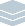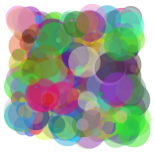Wolfram Archive
Wolfram Programming Lab is a legacy product.
All the same functionality and features, including access to Programming Lab Explorations, are available with Wolfram|One.
Start programming now. »
Try it now »
(no sign-in required)# Circlescapes

Make designs with random arrangements of circles.

Run the code to draw 100 random circles in a size 10 region. Try a different number of circles:

This draws a circle at coordinate {5,5}. PlotRange specifies the size of the drawing area:

Graphics[Circle[{5, 5}], PlotRange -> {{0, 10}, {0, 10}}]

Use RandomReal to draw circles at random positions.

This gives a random number between 0 and 10. Each time you run the code, you get a different number:

RandomReal

This gives a list of two random numbers:

RandomReal[10, 2]

This draws a circle at a random position. Each time you run the code, the circle is drawn in a different place:

Graphics[Circle[RandomReal[10, 2]], PlotRange -> {{0, 10}, {0, 10}}]

Use Table to draw lots of circles. Table makes lists of things.

This gives a list of 10 random coordinate positions:

Table[RandomReal[10, 2], {10}]

This draws 10 circles in random coordinate positions:

Graphics[Table[Circle[RandomReal[10, 2]], {10}], PlotRange -> {{0, 10}, {0, 10}}]

If you dont specify a PlotRange, the output will be just large enough to contain all the circles:

Graphics[Table[Circle[RandomReal[10, 2]], {10}]]

Graphics[Table[Circle[RandomReal[10, 2]], {100}]]

Use circles with random radii up to 1.5. Try ranges of radii other than 1.5:

This draws a circle at {5,5} with a radius of 1.5:

Graphics[Circle[{5, 5}, 1.5], PlotRange -> {{0, 10}, {0, 10}}]

Use RandomReal to specify a random radius. Each time you run the code, you get a different size of circle with a radius between 0 and 1.5:

Graphics[Circle[{5, 5}, RandomReal[1.5]], PlotRange -> {{0, 10}, {0, 10}}]

Make both the positions and the radii of the circles random:

Graphics[Table[Circle[RandomReal[10, 2], RandomReal[1.5]], {100}]]

Graphics[Table[Circle[RandomReal[10, 2], RandomReal[1.5]], {100}]]

Use random colors, and use disks instead of circles. Try numbers of disks other than 100:

Use Disk instead of Circle to get a filled-in shape:

Graphics[Disk[{5, 5}]]

Make the disk red:

Graphics[{Red, Disk[{5, 5}]}]

Make the disk a random color. Each time you run the code, you get a different color of disk:

Graphics[{RandomColor[], Disk[{5, 5}]}]

Make disks with random colors, random positions, and random sizes:

Graphics[Table[{RandomColor[], Disk[RandomReal[10, 2], RandomReal[1.5]]}, {100}]]

Graphics[Table[{RandomColor[], Disk[RandomReal[10, 2], RandomReal[1.5]]}, {100}]]

Make each disk have 50% opacity. Try different opacities:

Add Opacity[.5] to make the disks 50% opaque (which is 50% transparent):

Graphics[Table[{RandomColor[], Opacity[.5], Disk[RandomReal[10, 2], RandomReal[1.5]]}, {100}]]

Graphics[Table[{RandomColor[], Opacity[.5], Disk[RandomReal[10, 2], RandomReal[1.5]]}, {100}]]

Share ItMake a website that gives a different circlescape each time its visited:

Deploy the circlescape code to the Wolfram Cloud where anyone with a browser can use it:

CloudDeploy[ Delayed[ExportForm[ Graphics[ Table[{RandomColor[], Opacity[.5], Disk[RandomReal[10, 2], RandomReal[1.5]]}, {100}]], "PNG"]], "Permissions" -> "Public" ]

Click the link in the output to visit the site. Refresh the page in your browser to get a new circlescape.